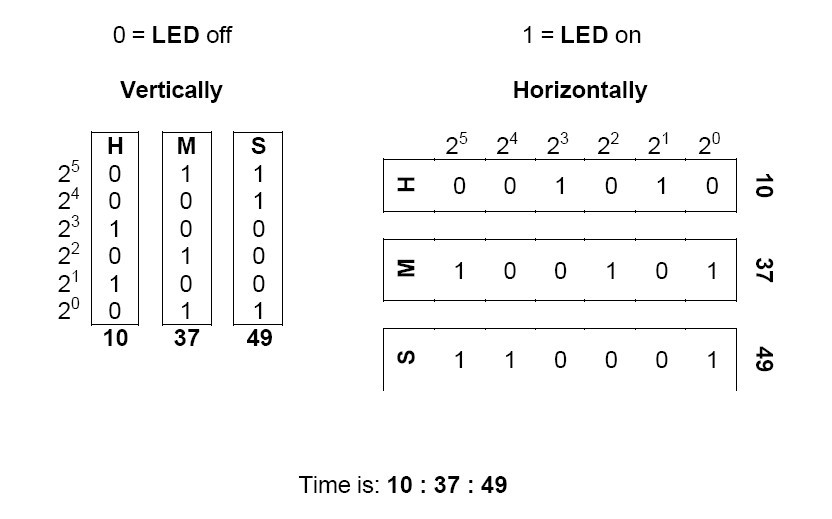# 2725. Binary Clock

A binary clock is a clock which displays traditional sexagesimal time (military format) in a binary format. The most common binary clock uses three columns or three rows of LEDs to represent zeros and ones. Each column (or row) represents a time-unit value.

When three columns are used (vertically), the bottom row in each column represents 1 (or 2^0), with each row above representing higher powers of two, up to 2^5 (or 32). To read each individual unit (hours, minutes or seconds) in the time, the user adds the values that each illuminated LED represents, and then reads the time from left to right. The first column represents the hour, the next column represents the minute, and the last column represents the second.

When three rows are used (horizontally), the right column in each row represents 1 (or 2^0), with each column left representing higher powers of two, up to 2^5 (or 32). To read each individual unit (hours, minutes or seconds) in the time, the user adds the values that each illuminated LED represents, and then reads the time from top to bottom. The top row represents the hour, the next row represents the minute, and the bottom row represents the second.

For example:For this problem you will read a time in sexagesimal time format, and output both the vertical and horizontal binary clock values. The output will be formed by concatenating together the bits in each column (or row) to form two 18 character strings of 1’s and 0’s as shown below.

10:37:49 would be written vertically as 011001100010100011 and horizontally as

001010100101110001.

### 输入格式

The first line of input contains a single integer N, (1 <= N <= 1000) which is the number of data sets that follow. Each data set consists of a single line of input containing the time in sexagesimal format.

### 输出格式

For each data set, you should generate one line of output with the following values: The data set number as a decimal integer (start counting at one), a space, the binary time in vertical format (18 binary digits), a space and the binary time in horizontal format (18 binary digits).

### 样例

Input
2
10:37:49
00:00:01

Output
1 011001100010100011 001010100101110001
2 000000000000000001 000000000000000001


48 人解决，52 人已尝试。

60 份提交通过，共有 77 份提交。

2.4 EMB 奖励。